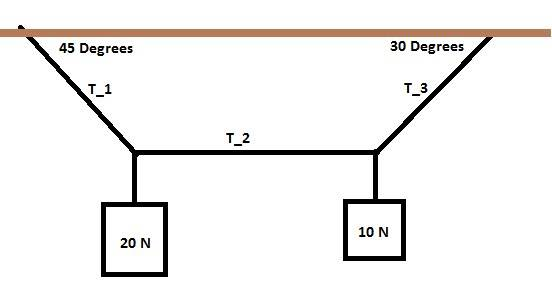# Vector Tension Problem

Homework Statement

2 weights of 20 N and 10 N are hung from 3 ropes. Find the tension of T1, T2, and T3:The attempt at a solution

If I designate one of the points as the origin, I get:

$$T_1sin45+T_2sin0+T_3sin30=30 N$$

and

$$T_2cos0+T_3cos30-T_1sin45=0$$

I'm having trouble finding a third equation... if those two are even right in the first place.

Try and stick to separating the forces into x and y components, at least mentally.

If you think about the forces that only act in the vertical direction for each weight separately you should be able to pretty much read off the values for T1 and T3, just use one of those to get you on to the shared tension.

You have four equations at your disposal, both axes for both weights.

Try and stick to separating the forces into x and y components, at least mentally.

If you think about the forces that only act in the vertical direction for each weight separately you should be able to pretty much read off the values for T1 and T3, just use one of those to get you on to the shared tension.

You have four equations at your disposal, both axes for both weights.

Sorry... I don't understand what you're saying. I don't know how to separate it into components with a system with more than one weight.

Sorry I thought that might be the problem,

At each junction of wires you can draw a little force diagram like this.

You can create four equations by focusing on only one axis at a time.

First one, this is only the force that acts parallel to the y-axis w/ the left knot at it's center.

$$\sum F_{y} = T_{1}Sin(45) -20 N. = 0$$